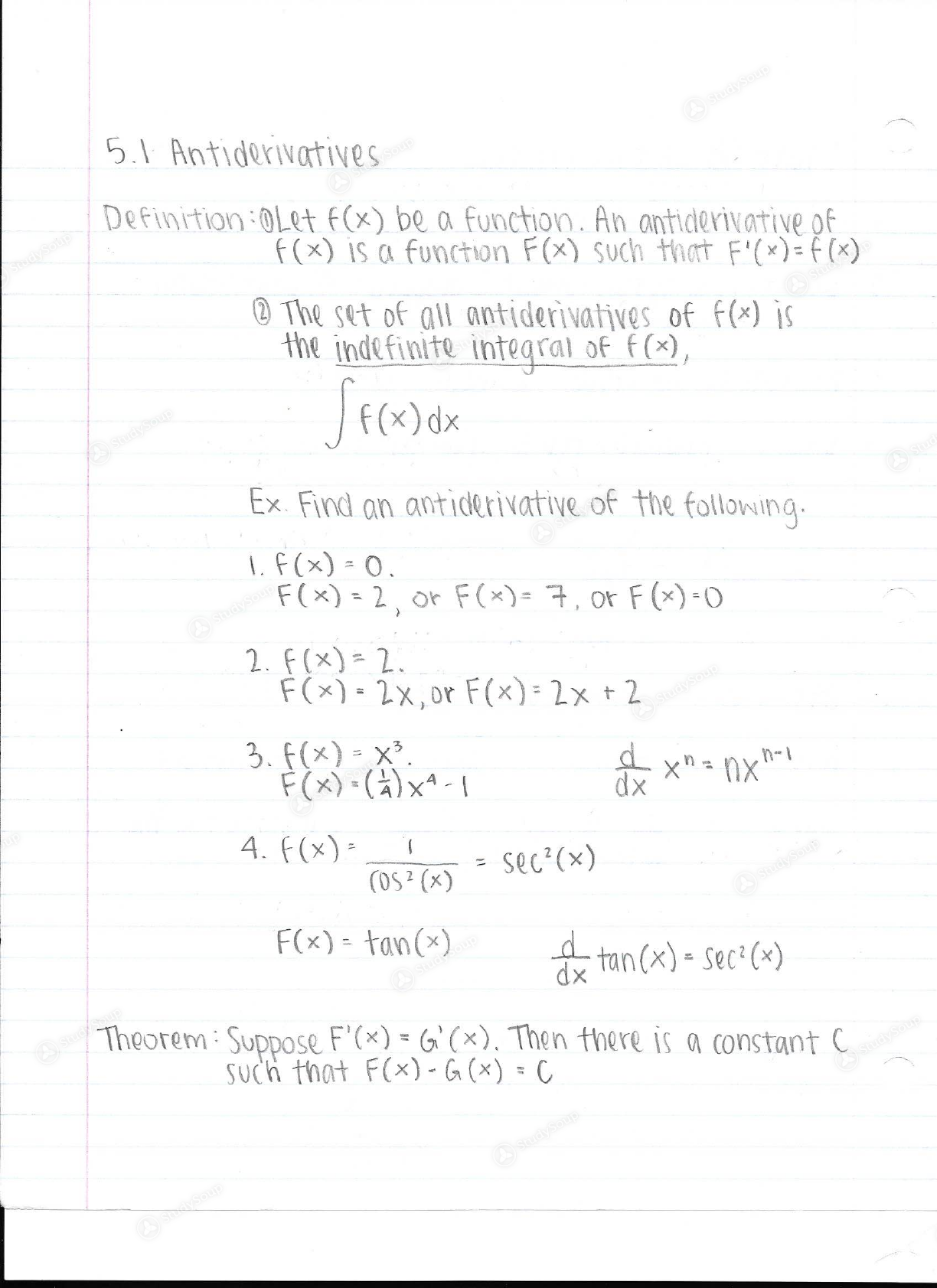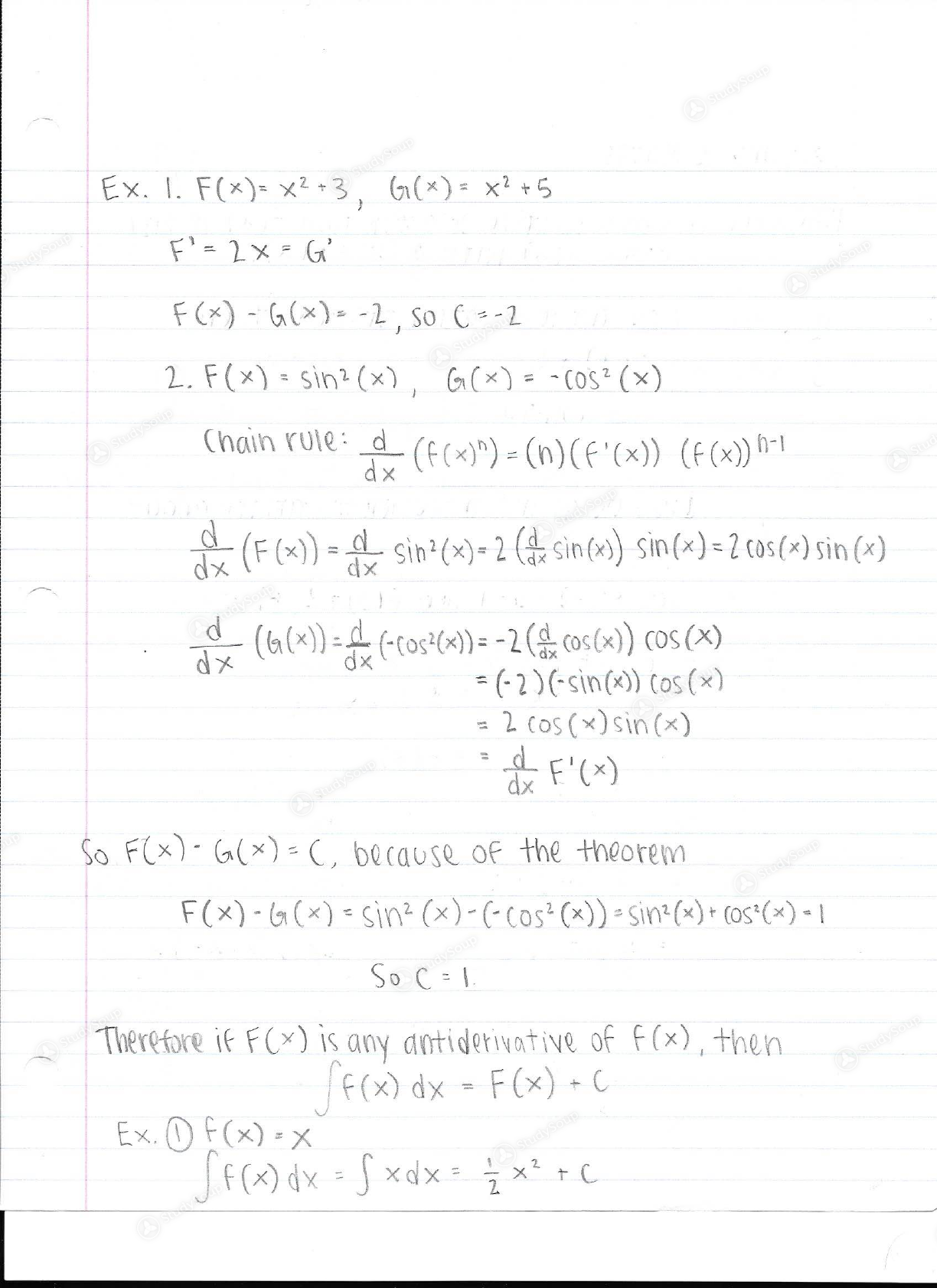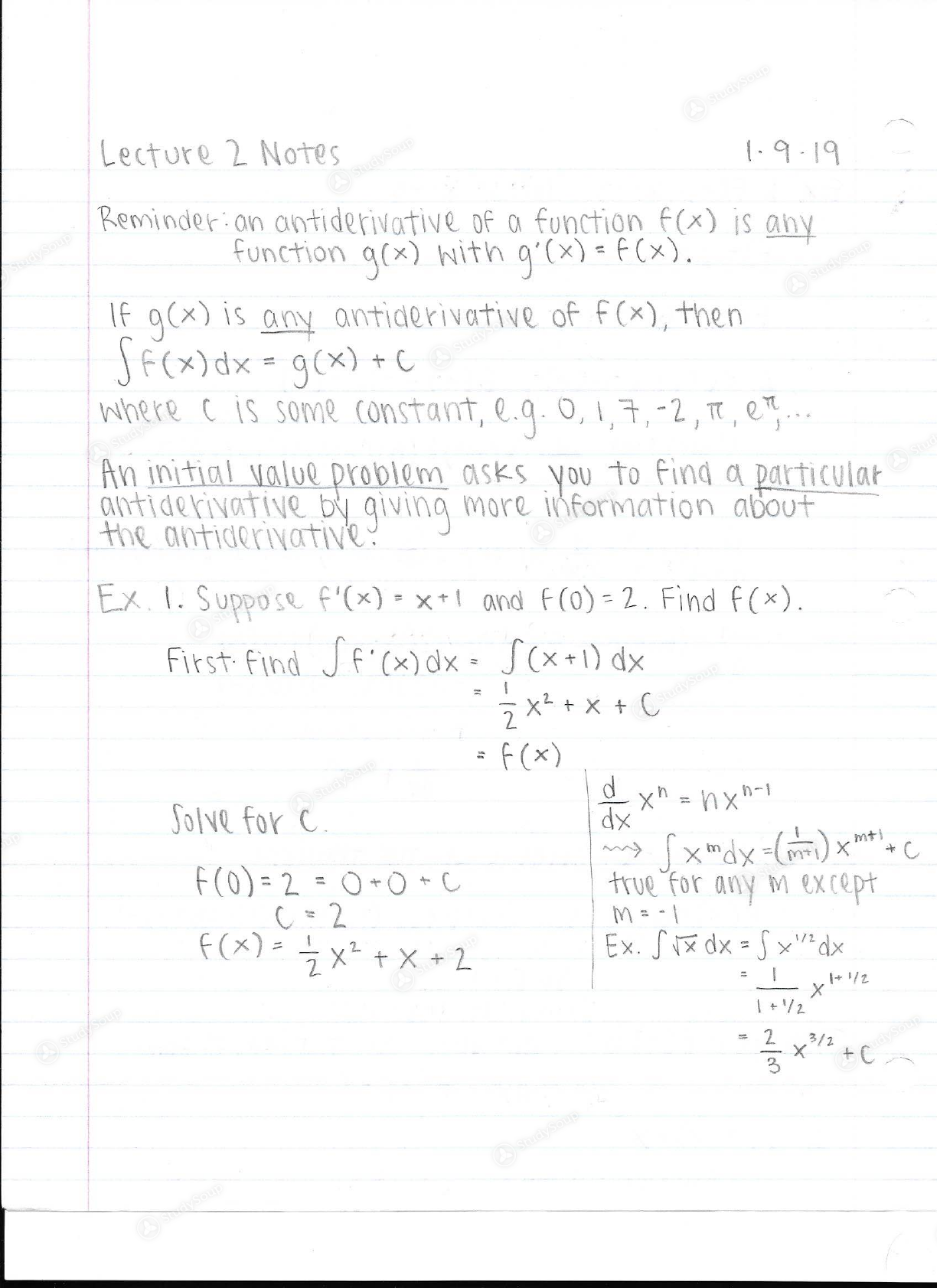Limited time offer 20% OFF StudySoup Subscription details

# UCR - MATH 009B - Math9B Week 1 Notes - Class Notes

### Created by: jewel.alexis Elite Notetaker

> > > > UCR - MATH 009B - Math9B Week 1 Notes - Class Notes
0 5 3 9 ReviewsJoin more than 18,000+ college students at University of California Riverside who use StudySoup to get ahead
##### Uploaded: 01/14/2019
48 Pages 52 Views 41 Unlocks
• Better Grades Guarantee
• 24/7 Homework help
• Notes, Study Guides, Flashcards + More!
##### Uploaded: 01/14/2019

Unformatted text preview: 5.1 Antiderivatives Definition :OLet f(x) be a function. An antiderivative of f(x) is a function F(x) such that F'(x)=f(x) 2 The set of all antiderivatives of f(x) is the indefinite integral of f(x), f(x) dx Ex. Find an antiderivative of the following. 1. F(x) - 0. F(x) = 2 or F(x)= 7, or F(x)=0 2. F(x)- 2. F(*). 2x,or F(x) = 2x + 2 3. f(x) - X?. F(x)- x- - X"=0X1 4. F(x) och 60 = secas F(x) = tan(x) =tan(x) = Sec?(*) Theorem : Suppose F'(x)=G(). Then there is a constant c such that F(x)-G(*) - CEx. I. F(x)=x2-3, 6(x) = x2 +5 F'- 2x-G F(x) - G(x)= -2,50 C --2 2. F(x) = sin2(x), G(X) = -(052 (x) Chain rule: (f(x)") = (n) (F'(x)) (f(x)) h-1 di (F (x) - a sin?(x)= 2 C. since) sin(x)=2cos() sin (4) ho (6(x)) = f(-Cos?(x) = -26cos(x)) cos(x) = (-2)(-sin(x)) Cos (x) = 2 cos(x) sin(x) . F'(x) So F(x)=G(*) - C, because of the theorem F(x)-G(*) - Sin? (x)-(-Cos? (x) - Sin?(x) + (05(x) - 1 SOC=1 Therefore if F(x) is any antiderivative of f(x), then F(x) dx - F(x) + Ex. Of(x) = x Sf(x) dx = 5 xdx = { x +CLecture 2 Notes 1.9.19 Reminder an antiderivative of a function f(x) is any function g(x) with g'(x) = f(x). If g(x) is any antiderivative of f(x), then SF(x) dx = g(x) + C where c is some constant, l.9.0.1,7,-2, TT, em An initial value problem asks you to find a particular antiderivative by giving more information about the antiderivative: Ex. I. Suppose f'(x) = x+1 and FCO) = 2. Find f(x). First. Find SF"(x) dx = S(x+1) dx 2 x2 + x + c f(x) Solve for C. x"=hx- my xmdy-() xmtlic f(0) = 2 = 0 O C true for any except C-2 f(x)= x2 + x + 2 Ex. Sva dx = Sxdx M 2 X +0